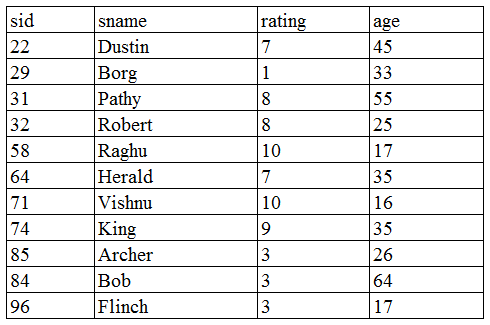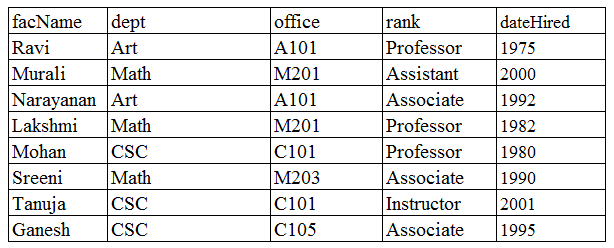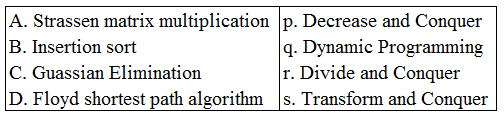# ISRO Scientist or Engineer Computer Science Dec 2017

Instructions

For the following questions answer them individually

Question 11

# Consider the schemaSailors(sid, sname, rating, age) with the following dataFor the querySELECT S.rating, AVG(S.age) AS avgage FROM Sailors SWhere S.age $$\ge$$ 18GROUP BY S.ratingHAVING 1 $$<$$ (SELECT COUNT($$*$$) FROM Sailors S2 where S.rating $$=$$ S2.rating)The number of rows returned isQuestion 12

# Consider a table that describes the customers :Customers(custid, name, gender, rating)The rating value is an integer in the range 1 to 5 and only two values (male and female) are recorded for gender. Consider the query “how many male customers have a rating of 5”? The best indexing mechanism appropriate for the query isQuestion 13

# Consider the following schema :Sailors(sid,sname,rating,age)Boats(bid,bname, colour)Reserves(sid,bid,day)Two boats can have the same name but the colour differentiates them.The two relations$$\rho(Tempsids, (\pi_{sid, bid}Reserves)/(\pi_{bid }(\sigma_{bname = 'Ganga'} Boats)))$$,$$\pi_{sname}(Tempsids \bowtie Sailors)$$If / is division operation, the above set of relations represents the queryQuestion 14

# Type IV JDBC driver is a driverQuestion 15

# Consider the following table : Faculty(facName, dept, office, rank, dateHired)(Assume that no faculty member within a single department has same name. Each faculty member has only one office identified in office). 3NF refers to third normal form and BNCFrefers to Boyce-Codd normal form Then Faculty isQuestion 16

# Consider the following query :SELECT E.eno, COUNT(*)FROM Employees EGROUP BY E.enoIf an index on eno is available, the query can be answered by scanning only the index ifQuestion 17

# If C is a skew-symmetric matrix of order n and X is $$n \times 1$$ column matrix, then $$X^T CX$$ is aQuestion 18

# Consider the recurrence equation$$T(n) = \begin{cases}2T(n - 1), & if n> 0\\1 & otherwise\end{cases}$$Then T(n) is (in big O order)Question 19

# Consider the programvoid function (int n) { int i, j, count = 0; for (i = n/2; i <= n; i++) for (j = 1; j <= n; j = j * 2) count++;}The complexity of the program isQuestion 20

# Match the following and choose the correct answer for the order A,B,C,DOR